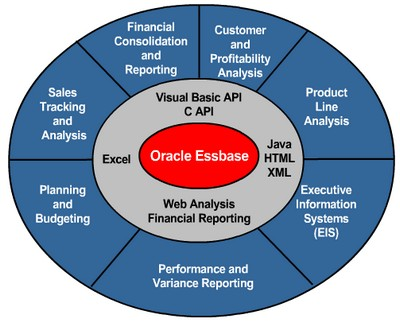# Essbase - Functions

Functions are predefined routines that perform specialized calculations and return :

• sets of members
• or sets of data values.

## Type of functions

The Essbase functions include more than 100 predefined routines to extend the calculation capabilities of Essbase. Essbase supports the following functions:

• Boolean functions, which provide a conditional test by returning a TRUE or FALSE value
• Mathematical functions, which perform specialized mathematical calculations
• Relationship functions, which look up data values within a database during a calculation based on the position of the current member
• Range functions, which declare a range of members as an argument to another function or to a command
• Financial functions, which perform specialized financial calculations
• Member set functions, which are based on a specified member and which generate lists of members
• Allocation functions, which allocate values that are input at a parent level across child members
• Forecasting functions, which manipulate data for the purpose of smoothing data, interpolating data, or calculating future values
• Statistical functions, which calculate advanced statistics
• Date and time functions, which use date and time characteristics in calculation formulas
• Calculation mode functions, which specify the calculation mode that Essbase uses to calculate a formula

## Documentation / Reference

Discover MoreEssbase - Calculations

Essbase includes powerful calculation features for demanding analytic requirements. A rich library of functions makes it easy to define advanced and sophisticated business logic and relationships. Essbase...Essbase - Formulas

Formulas calculate relationships between member in the database outline. You can : apply formulas to members in the outline, or place formulas in a calculation script. This topic explains how TBC...